Latest SSC jobs   »   Histogram   »   Histogram

# Histogram Definition, Graph, Chart, Formula and Statistics

## Histogram Definition

A Histogram is a graphical representation of data using rectangles that shows the frequency of data in successive intervals of equal sizes. The distribution of data is shown using rectangles adjacent to each other in the histogram. The height of rectangles in the histogram is proportional to the corresponding frequencies of similar or different classes. The histogram is a technique to represent huge data conveniently. It is used to summarize or represent large data at given intervals.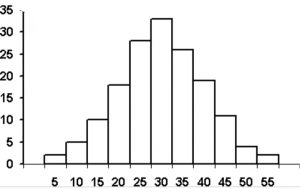## Histogram Graph

A histogram graph is a representation of data on a bar graph. It shows data in visual form using bar graphs with the help of rectangles. On the graph paper, the data is represented using the following rules.

• The number range is shown on the horizontal axis.
• The vertical axis (frequency) represents the amount of data that is present or repeats in each range.

It is a representation of a range of outcomes in column formation along the x-axis. while the number count or multiple occurrences in the data for each column is represented on the y-axis. You can understand the histogram graph easily through the example discussed below.

A teacher wanted to compare the performance of two groups of students in a Physics test of 100  marks. Looking at their performances, he found that a few students got under 20 marks and a few got 70 marks or above. So he decided to group them into intervals of varying sizes as shown below:

0 – 20, 20 – 30, 30 – 40, 40 – 50, 50 – 60, 60 – 70, 70 – 100

Then the teacher formed a table

 Marks Number of Students 0−200−20 77 20−3020−30 1010 30−4030−40 1010 40−5040−50 2020 50−6050−60 2020 60−7060−70 1515 7070-above 88 Total 90

Solution: We need to make certain changes in the lengths of the rectangles so that the areas should be proportional to the frequencies.

The steps to be followed are listed below:

1. First, select the class interval with the minimum class size.
2. Then lengths of the rectangles need to modify to be proportionate to the class size.

When the class size is 20 the length of the rectangle is 77. So, when the class size is 10 the length of the rectangle will be 7/20 × 10 = 3.5

Then the changes in the table occurred. Now the table will be as shown below:

 Marks Frequency Width of the class Length of the rectangle 0−200−20 77 2020 720×10=3.5720×10=3.5 20−3020−30 1010 1010 1010×10=101010×10=10 30−4030−40 1010 1010 1010×10=101010×10=10 40−5040−50 2020 1010 2010×10=202010×10=20

Since we have calculated these lengths for an interval of 10 marks in each case, then the length will be the “proportion of students per 10 marks interval”.

So, the correct histogram formed with varying widths is given in the below figure.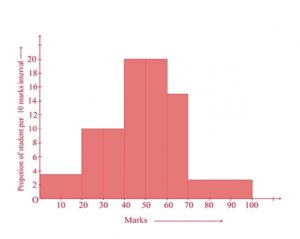## Histogram Formula

In the histogram, we need to find the area of rectangles to get the required results. The formula used to calculate the area of the rectangle with the given data is shown below.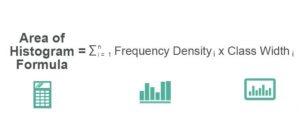## Histogram Statistics

In statistics, the histogram is used mostly to represent the data in an organized form. The histogram is used to represent the unorganized data in a convenient form. It is used in various applications for daily life purposes as well as in mathematics and science.

## Histogram Chart

A histogram is a chart that groups numeric data into intervals or bins, displaying the bins as segmented columns. They’re used to depict the distribution of a dataset: how often values fall into this range. A diagrammatical representation of a histogram chart is shown below.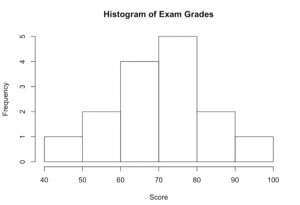## Histogram vs Bar Graph

• A bar graph is the graphical representation of the data using rectangular bars where the length of each bar is proportional to its values.
• A histogram is the graphical representation of data where data is grouped into continuous number ranges and each range corresponds to a vertical bar.
 Bar graph Histogram The bar graph is the graphical representation of categorical data Bar graph is the graphical representation of grouped data in a continuous manner There is equal space between each pair of consecutive bars There is no space between the consecutive bars The height of the bars shows the frequency and the width gap is zero The frequency of the data is represented by the area of rectangles.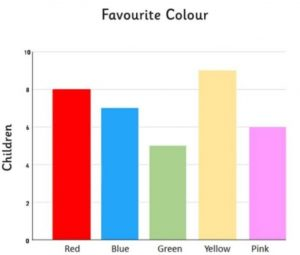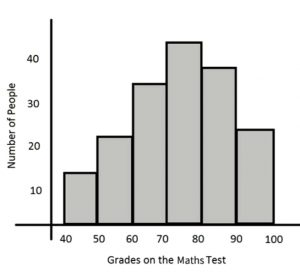Histogram Example

Example: 1 Consider the following histogram that represents the weights of 34 newborn babies in a government hospital. If the children weighing between 6.5 lb to 8.5 lb are considered healthy, then find the percentage of the healthy children in this hospital.

Solution:  Firstly, We have to find the number of children weighing between 4.4 lb to 6.6 lb. From the given histogram, the number of children weighing between:

6.5 lb – 7.5 lb = 10
7.5 lb – 8.5 lb = 18
Therefore, the number of children weighing between 6.5 lb to 8.5 lb = (10+18=28). The total number of children in the hospital = 34. Hence, the required percentage is 28/34 × 100 = approx 83%. ∴ Required percentage = 83%.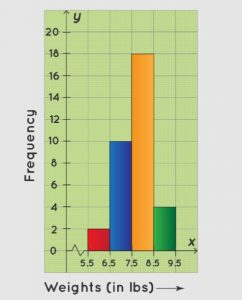We hope this information will be helpful for you, and for more study notes like this stay connected with us. If you want to read this article in Hindi please click on the link Provided below.

Histogram Definition, Graph, Chart, Formula and Statistics

Histogram: FAQs

Que.1 What is histogram?

Ans – Histogram is the graphical representation of data using rectangular bars.

Que.2 What is a bar graph?

Ans – A bar graph is the graphical representation of categorical data.

#### Congratulations!General Awareness & Science Capsule PDF•Elementary Statistics - Problems, Questi...
•What is Frequency Polygon? Statistics, G...
•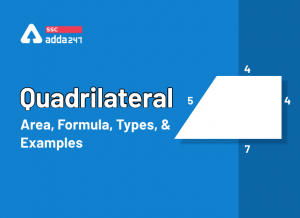Quadrilateral Area, Formula, Types, Prop...
•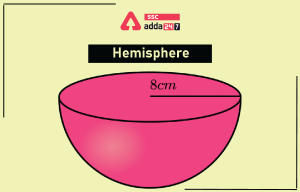Hemisphere Definition, Volume, Formula, ...